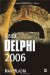Arithmetic Operators and Expressions

Delphi has six binary and two unary arithmetic operators.

Binary Arithmetic Operators

Binary arithmetic operators in Delphi are:

• Subtraction (–)

• Multiplication (*)

• Integer division (div)

• Real division (/)

• Remainder (mod)

Delphi has two different operators for division. The div operator is meant to be used with integer values and the / operator is meant to be used with floating-point values. The div operator returns an integer value rounded toward the smaller value, and the / operator always returns a real value, as shown in Listing 2-10.

Listing 2-10: Real and integer divisionvar   x: Integer;   d: Double; begin   x := 11 div 2;   d := 11 / 2;   WriteLn(x);   { Displays 5 }   WriteLn(d:0:2);   { Displays 5.50 }   ReadLn; end.The mod operator is used to return the remainder of an integer division (see Listing 2-11). If you're a beginning programmer you may not find this operator interesting, but there are a number of situations where it helps significantly. For example, you can use the mod operator when you want to do something after a specific interval or to easily find out if a specific year is a leap year.

Listing 2-11: The mod operatorvar   x: Integer;   y: Integer;   start: Integer; begin   start := 17;   x := start div 3;   y := start mod 3;   WriteLn(x); { Displays 5 }   WriteLn(y); { Displays 2 }   ReadLn; end.Unary Arithmetic Operators

Delphi has two unary operators: unary + and unary –. The unary plus is not used very much (if at all) since its usage always results in the original value (see Listing 2-12).

Listing 2-12: The unary plus operatorvar   x: Integer; begin   x := 20;   WriteLn(+x);   x := -20;   WriteLn(+x);   ReadLn; end.On the other hand, the unary minus operator always changes the selected value (inverts the sign) and can sometimes be very handy and result in pretty clean and fast code.

Expressions

Each operator in Delphi has its own precedence — the precedence of execution in an expression. Arithmetic operators that have the highest precedence are *, /, div, and mod. Arithmetic operators with lower precedence are the + and – operators.

When Delphi reads an expression, it reads the expression from left to right but evaluates operators according to operator precedence. When Delphi reads the expression 10 + 20 * 5, it will first multiply the numbers 20 and 5 and then add the resulting value to the first operand. If the expression only contains operators of the same precedence, they will be evaluated as they appear in the expression.

You can override the precedence of an operation by enclosing it in parentheses. In the expression (1 + 2) * 3, the operation in parentheses will be evaluated first.

Listing 2-13: Arithmetic operator precedencevar   x: Integer;   y: Integer; begin   x := 20 * 9 + 3;   y := 20 * (9 + 3);   WriteLn(x);      { Displays 183 }   WriteLn(y);      { Displays 240 }   ReadLn; end.Inside Delphi 2006 (Wordware Delphi Developers Library)
ISBN: 1598220039
EAN: 2147483647
Year: 2004
Pages: 212